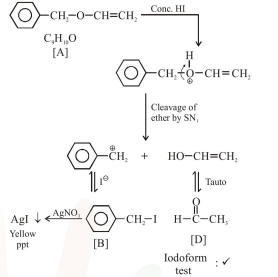# An organic compound 'A' ( C9H10O ) when treated with conc.

Question:

An organic compound ' $\mathrm{A}^{\prime}\left(\mathrm{C}_{9} \mathrm{H}_{10} \mathrm{O}\right)$ when treated with conc. HI undergoes cleavage

to yield compounds ' $B$ ' and ' $C$ '. 'B' gives yellow precipitate with $\mathrm{AgNO}_{3}$ where as ' $\mathrm{C}$ ' tautomerizes to 'D', 'D' gives

positive idoform test. 'A' could be :

1.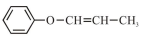2.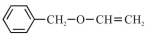3.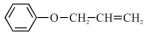4.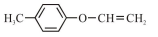Correct Option: , 2

Solution: